Скачать презентацию Lecture 11 Heat Engines Ch 4 A heat

dc616074235428a876b596daa20937b3.ppt

• Количество слайдов: 19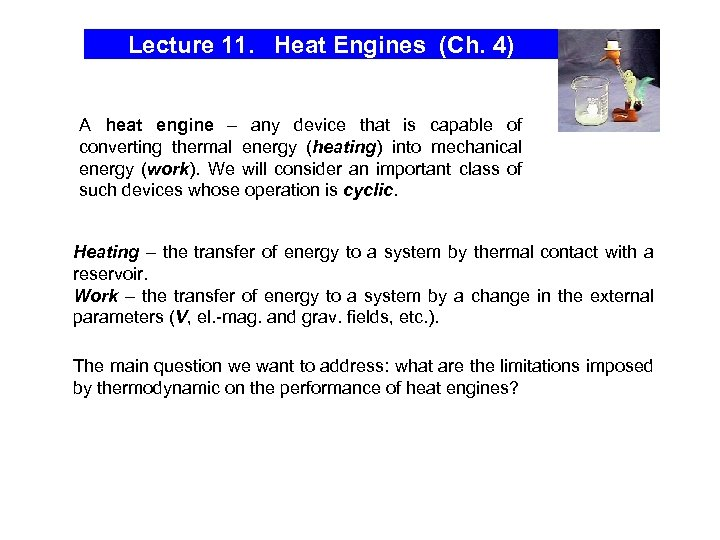Lecture 11. Heat Engines (Ch. 4) A heat engine – any device that is capable of converting thermal energy (heating) into mechanical energy (work). We will consider an important class of such devices whose operation is cyclic. Heating – the transfer of energy to a system by thermal contact with a reservoir. Work – the transfer of energy to a system by a change in the external parameters (V, el. -mag. and grav. fields, etc. ). The main question we want to address: what are the limitations imposed by thermodynamic on the performance of heat engines?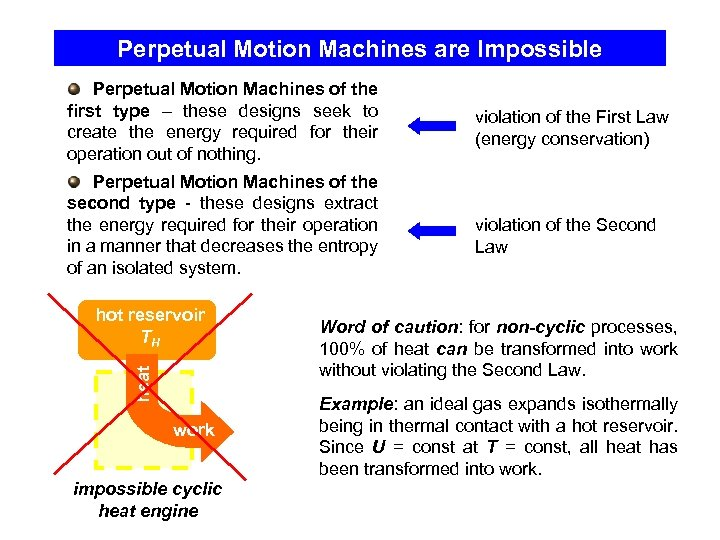Perpetual Motion Machines are Impossible Perpetual Motion Machines of the first type – these designs seek to create the energy required for their operation out of nothing. Perpetual Motion Machines of the second type - these designs extract the energy required for their operation in a manner that decreases the entropy of an isolated system. heat hot reservoir TH work impossible cyclic heat engine violation of the First Law (energy conservation) violation of the Second Law Word of caution: for non-cyclic processes, 100% of heat can be transformed into work without violating the Second Law. Example: an ideal gas expands isothermally being in thermal contact with a hot reservoir. Since U = const at T = const, all heat has been transformed into work.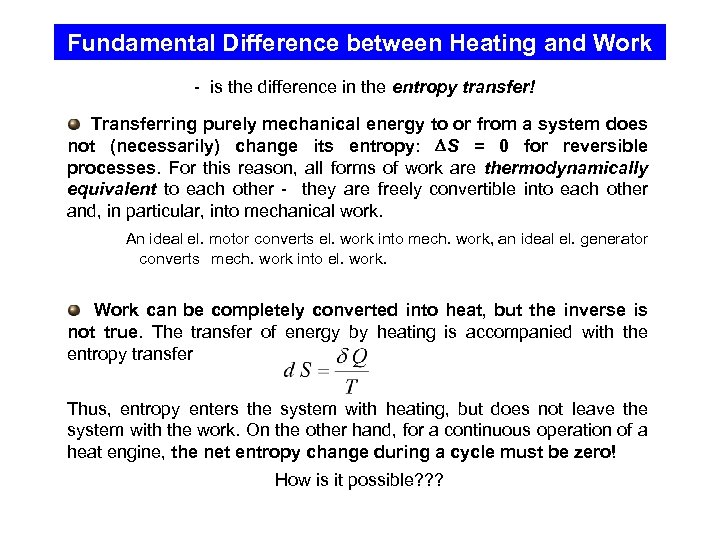Fundamental Difference between Heating and Work - is the difference in the entropy transfer! Transferring purely mechanical energy to or from a system does not (necessarily) change its entropy: S = 0 for reversible processes. For this reason, all forms of work are thermodynamically equivalent to each other - they are freely convertible into each other and, in particular, into mechanical work. An ideal el. motor converts el. work into mech. work, an ideal el. generator converts mech. work into el. work. Work can be completely converted into heat, but the inverse is not true. The transfer of energy by heating is accompanied with the entropy transfer Thus, entropy enters the system with heating, but does not leave the system with the work. On the other hand, for a continuous operation of a heat engine, the net entropy change during a cycle must be zero! How is it possible? ? ?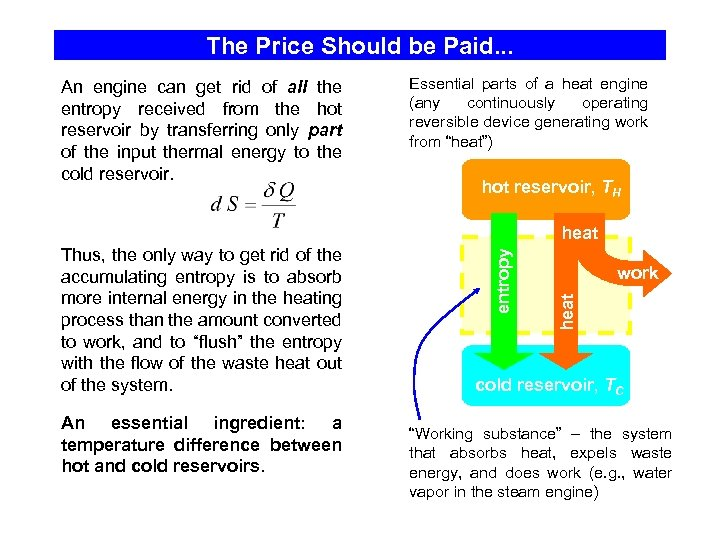The Price Should be Paid. . . An engine can get rid of all the entropy received from the hot reservoir by transferring only part of the input thermal energy to the cold reservoir. Essential parts of a heat engine (any continuously operating reversible device generating work from “heat”) hot reservoir, TH An essential ingredient: a temperature difference between hot and cold reservoirs. work heat Thus, the only way to get rid of the accumulating entropy is to absorb more internal energy in the heating process than the amount converted to work, and to “flush” the entropy with the flow of the waste heat out of the system. entropy heat cold reservoir, TC “Working substance” – the system that absorbs heat, expels waste energy, and does work (e. g. , water vapor in the steam engine)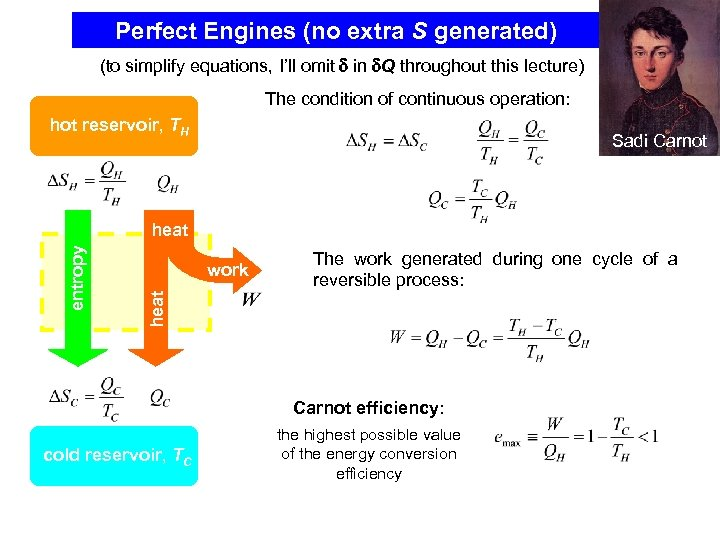Perfect Engines (no extra S generated) (to simplify equations, I’ll omit in Q throughout this lecture) The condition of continuous operation: hot reservoir, TH Sadi Carnot work The work generated during one cycle of a reversible process: heat entropy heat Carnot efficiency: cold reservoir, TC the highest possible value of the energy conversion efficiency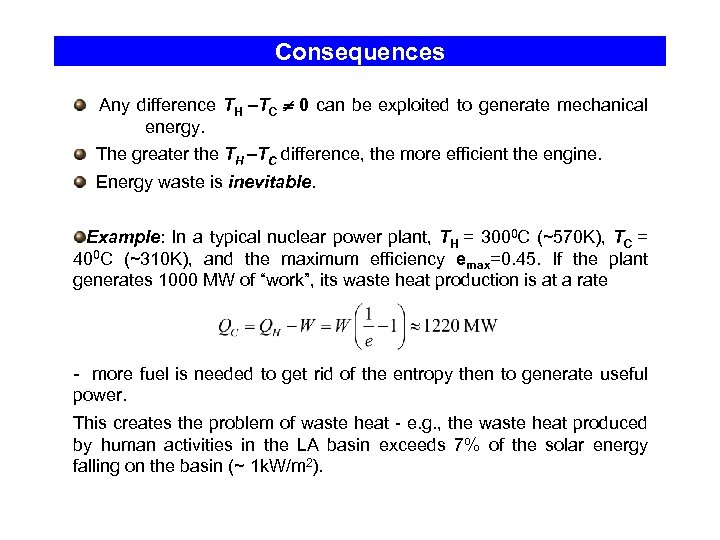Consequences Any difference TH –TC 0 can be exploited to generate mechanical energy. The greater the TH –TC difference, the more efficient the engine. Energy waste is inevitable. Example: In a typical nuclear power plant, TH = 3000 C (~570 K), TC = 400 C (~310 K), and the maximum efficiency emax=0. 45. If the plant generates 1000 MW of “work”, its waste heat production is at a rate - more fuel is needed to get rid of the entropy then to generate useful power. This creates the problem of waste heat - e. g. , the waste heat produced by human activities in the LA basin exceeds 7% of the solar energy falling on the basin (~ 1 k. W/m 2).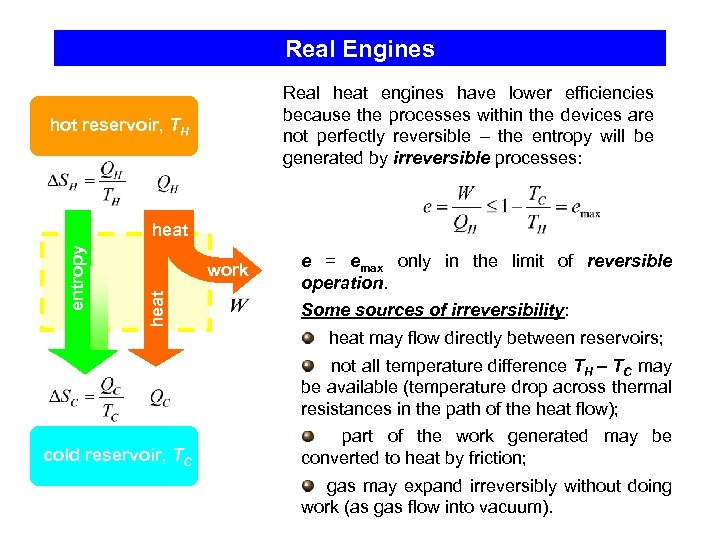Real Engines Real heat engines have lower efficiencies because the processes within the devices are not perfectly reversible – the entropy will be generated by irreversible processes: hot reservoir, TH work heat entropy heat e = emax only in the limit of reversible operation. Some sources of irreversibility: heat may flow directly between reservoirs; not all temperature difference TH – TC may be available (temperature drop across thermal resistances in the path of the heat flow); cold reservoir, TC part of the work generated may be converted to heat by friction; gas may expand irreversibly without doing work (as gas flow into vacuum).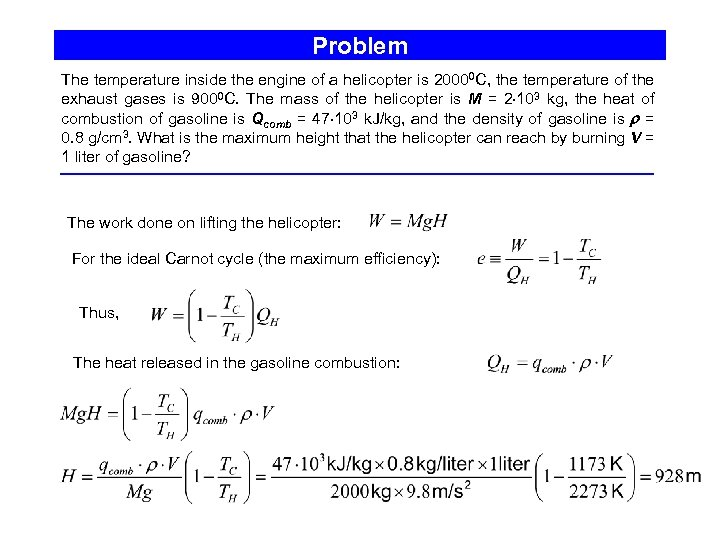Problem The temperature inside the engine of a helicopter is 20000 C, the temperature of the exhaust gases is 9000 C. The mass of the helicopter is M = 2 103 kg, the heat of combustion of gasoline is Qcomb = 47 103 k. J/kg, and the density of gasoline is = 0. 8 g/cm 3. What is the maximum height that the helicopter can reach by burning V = 1 liter of gasoline? The work done on lifting the helicopter: For the ideal Carnot cycle (the maximum efficiency): Thus, The heat released in the gasoline combustion: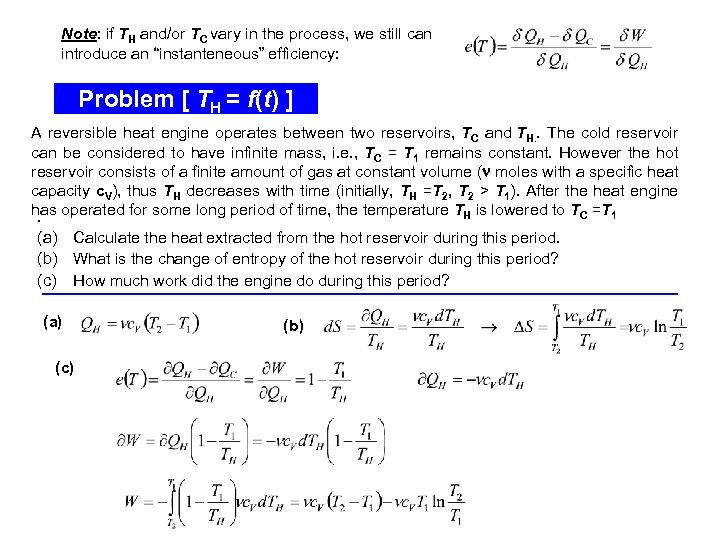Note: if TH and/or TC vary in the process, we still can introduce an “instanteneous” efficiency: Problem [ TH = f(t) ] A reversible heat engine operates between two reservoirs, TC and TH. . The cold reservoir can be considered to have infinite mass, i. e. , TC = T 1 remains constant. However the hot reservoir consists of a finite amount of gas at constant volume ( moles with a specific heat capacity c. V), thus TH decreases with time (initially, TH =T 2, T 2 > T 1). After the heat engine has operated for some long period of time, the temperature TH is lowered to TC =T 1. (a) Calculate the heat extracted from the hot reservoir during this period. (b) What is the change of entropy of the hot reservoir during this period? (c) How much work did the engine do during this period? (a) (c) (b)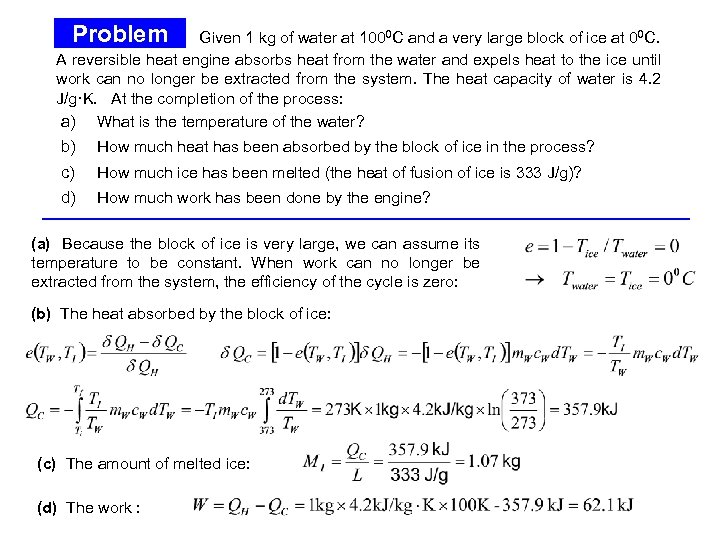Problem Given 1 kg of water at 1000 C and a very large block of ice at 00 C. A reversible heat engine absorbs heat from the water and expels heat to the ice until work can no longer be extracted from the system. The heat capacity of water is 4. 2 J/g·K. At the completion of the process: a) What is the temperature of the water? b) How much heat has been absorbed by the block of ice in the process? c) How much ice has been melted (the heat of fusion of ice is 333 J/g)? d) How much work has been done by the engine? (a) Because the block of ice is very large, we can assume its temperature to be constant. When work can no longer be extracted from the system, the efficiency of the cycle is zero: (b) The heat absorbed by the block of ice: Problem (cont. ) (c) The amount of melted ice: (d) The work :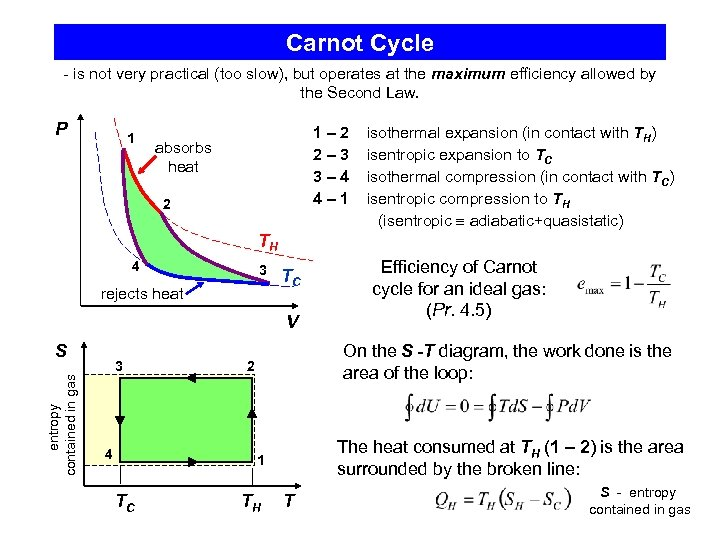Carnot Cycle - is not very practical (too slow), but operates at the maximum efficiency allowed by the Second Law. P 1 1– 2 2– 3 3– 4 4– 1 absorbs heat 2 isothermal expansion (in contact with TH) isentropic expansion to TC isothermal compression (in contact with TC) isentropic compression to TH (isentropic adiabatic+quasistatic) TH 4 3 rejects heat TC V entropy contained in gas S 3 4 On the S -T diagram, the work done is the area of the loop: 2 The heat consumed at TH (1 – 2) is the area surrounded by the broken line: 1 TC Efficiency of Carnot cycle for an ideal gas: (Pr. 4. 5) TH T S - entropy contained in gas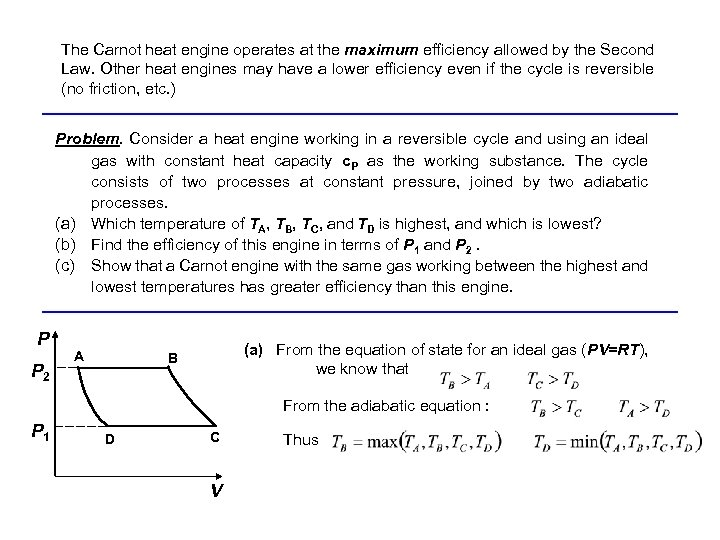The Carnot heat engine operates at the maximum efficiency allowed by the Second Law. Other heat engines may have a lower efficiency even if the cycle is reversible (no friction, etc. ) Problem. Consider a heat engine working in a reversible cycle and using an ideal gas with constant heat capacity c. P as the working substance. The cycle consists of two processes at constant pressure, joined by two adiabatic processes. (a) Which temperature of TA, TB, TC, and TD is highest, and which is lowest? (b) Find the efficiency of this engine in terms of P 1 and P 2. (c) Show that a Carnot engine with the same gas working between the highest and lowest temperatures has greater efficiency than this engine. P P 2 A (a) From the equation of state for an ideal gas (PV=RT), we know that B From the adiabatic equation : P 1 D C V Thus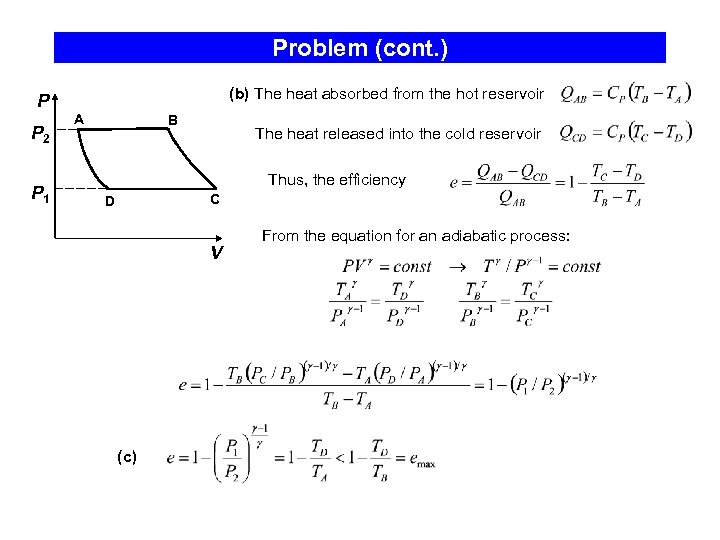Problem (cont. ) P P 2 P 1 (b) The heat absorbed from the hot reservoir A B The heat released into the cold reservoir Thus, the efficiency C D V (c) From the equation for an adiabatic process: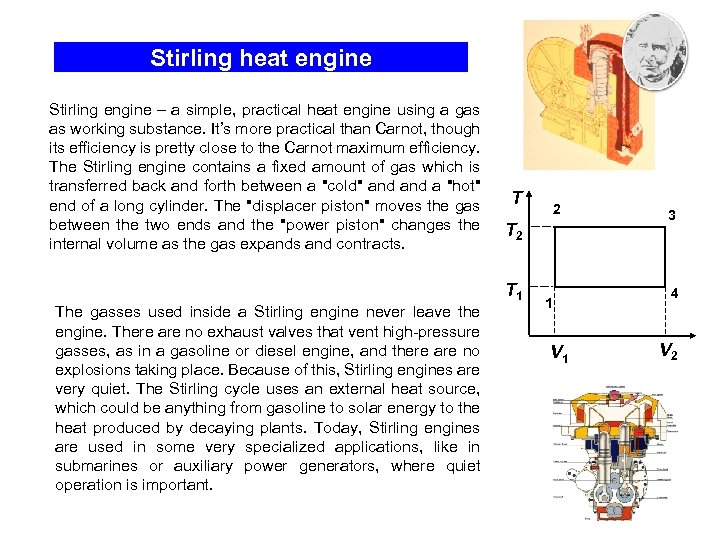Stirling heat engine Stirling engine – a simple, practical heat engine using a gas as working substance. It’s more practical than Carnot, though its efficiency is pretty close to the Carnot maximum efficiency. The Stirling engine contains a fixed amount of gas which is transferred back and forth between a "cold" and a "hot" end of a long cylinder. The "displacer piston" moves the gas between the two ends and the "power piston" changes the internal volume as the gas expands and contracts. The gasses used inside a Stirling engine never leave the engine. There are no exhaust valves that vent high-pressure gasses, as in a gasoline or diesel engine, and there are no explosions taking place. Because of this, Stirling engines are very quiet. The Stirling cycle uses an external heat source, which could be anything from gasoline to solar energy to the heat produced by decaying plants. Today, Stirling engines are used in some very specialized applications, like in submarines or auxiliary power generators, where quiet operation is important. T 2 T 1 1 V 1 3 4 V 2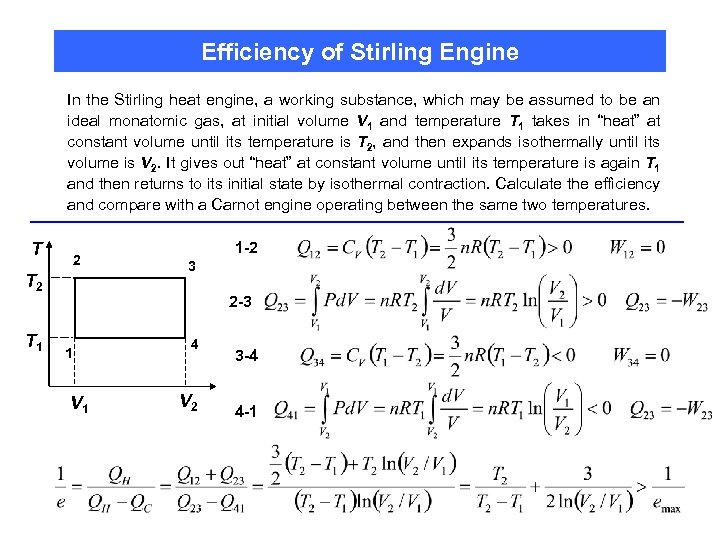Efficiency of Stirling Engine In the Stirling heat engine, a working substance, which may be assumed to be an ideal monatomic gas, at initial volume V 1 and temperature T 1 takes in “heat” at constant volume until its temperature is T 2, and then expands isothermally until its volume is V 2. It gives out “heat” at constant volume until its temperature is again T 1 and then returns to its initial state by isothermal contraction. Calculate the efficiency and compare with a Carnot engine operating between the same two temperatures. T 2 T 1 1 -2 3 2 -3 1 V 1 4 V 2 3 -4 4 -1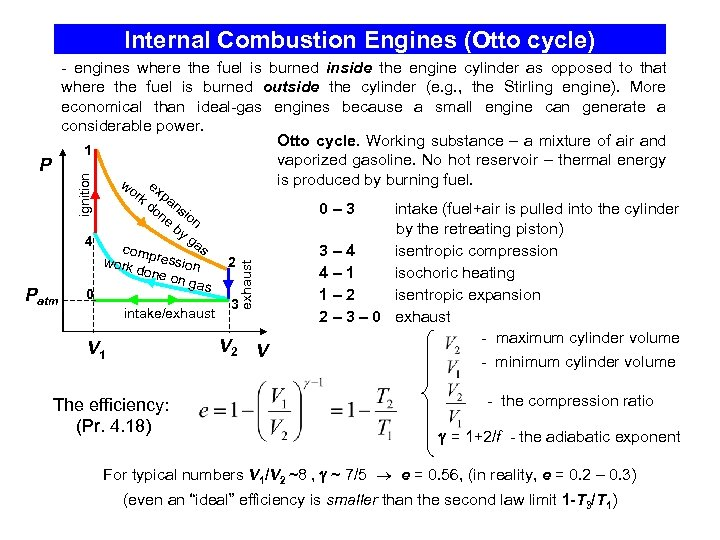Internal Combustion Engines (Otto cycle) ignition - engines where the fuel is burned inside the engine cylinder as opposed to that where the fuel is burned outside the cylinder (e. g. , the Stirling engine). More economical than ideal-gas engines because a small engine can generate a considerable power. Otto cycle. Working substance – a mixture of air and 1 vaporized gasoline. No hot reservoir – thermal energy P is produced by burning fuel. w e x k d pan on sio e by n ga 4 s comp ressi work 2 done on on ga s 0 3 intake/exhaust 0– 3 exhaust Patm or V 2 V 1 The efficiency: (Pr. 4. 18) V intake (fuel+air is pulled into the cylinder by the retreating piston) 3– 4 isentropic compression 4– 1 isochoric heating 1– 2 isentropic expansion 2 – 3 – 0 exhaust - maximum cylinder volume - minimum cylinder volume - the compression ratio = 1+2/f - the adiabatic exponent For typical numbers V 1/V 2 ~8 , ~ 7/5 e = 0. 56, (in reality, e = 0. 2 – 0. 3) (even an “ideal” efficiency is smaller than the second law limit 1 -T 3/T 1)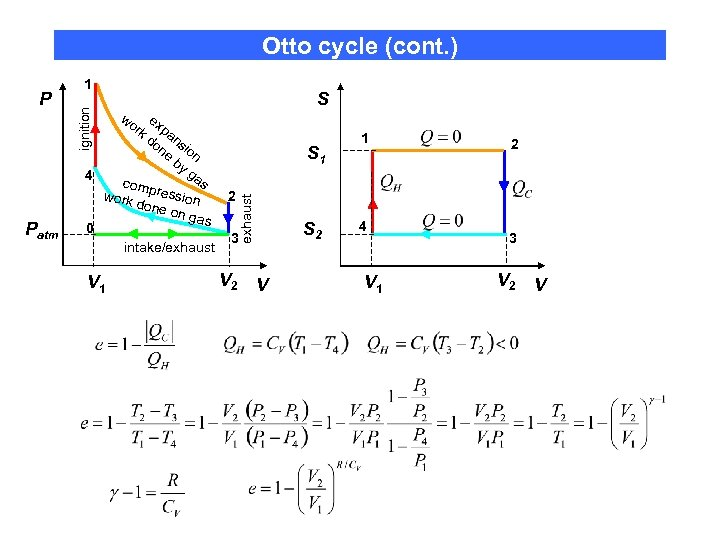Otto cycle (cont. ) S wo e rk xpa do ns ne ion by ga 4 s comp ressi work 2 done on on ga s 0 3 intake/exhaust S 1 exhaust Patm ignition P 1 V 2 S 2 V 1 4 V 1 2 3 V 2 V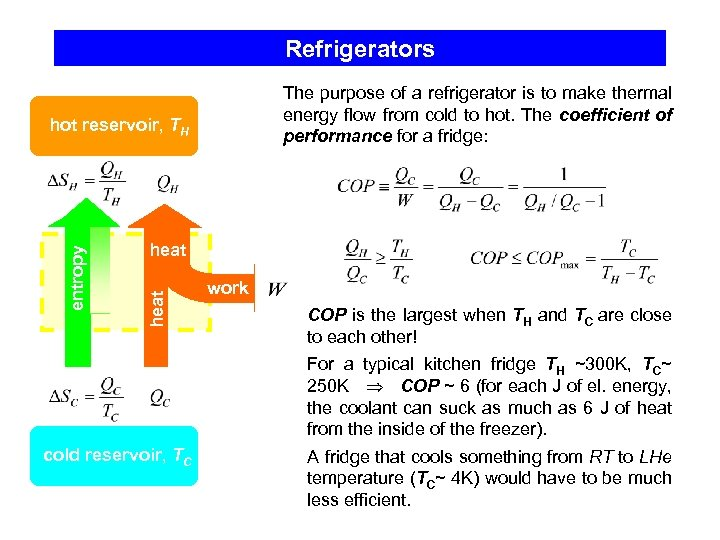Refrigerators The purpose of a refrigerator is to make thermal energy flow from cold to hot. The coefficient of performance for a fridge: heat entropy hot reservoir, TH work COP is the largest when TH and TC are close to each other! For a typical kitchen fridge TH ~300 K, TC~ 250 K COP ~ 6 (for each J of el. energy, the coolant can suck as much as 6 J of heat from the inside of the freezer). cold reservoir, TC A fridge that cools something from RT to LHe temperature (TC~ 4 K) would have to be much less efficient.Example: A “perfect” heat engine with e = 0. 4 is used as a refrigerator (the heat reservoirs remain the same). How much heat QC can be transferred in one cycle from the cold reservoir to the hot one if the supplied in one cycle work is W =10 k. J?